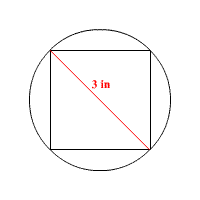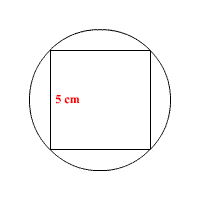# Squares Circumscribed by Circles

When a square is circumscribed by a circle , the diagonal of the square is equal to the diameter of the circle.

Example 1:

Find the side length $s$ of the square.The diagonal of the square is $3$ inches. We know from the Pythagorean Theorem that the diagonal of a square is $\sqrt{2}$ times the length of a side. Therefore:

$\begin{array}{l}s\sqrt{2}=3\\ s=\frac{3}{\sqrt{2}}=\frac{3\sqrt{2}}{2}\text{\hspace{0.17em}}\text{\hspace{0.17em}}\text{in}.\end{array}$

Example 2:

Find the area of the circle.First, find the diagonal of the square. Its length is $\sqrt{2}$ times the length of the side, or $5\sqrt{2}$ cm.

This value is also the diameter of the circle. So, the radius of the circle is half that length, or $\frac{5\sqrt{2}}{2}$ .

To find the area of the circle, use the formula $A=\pi {r}^{2}$ .

$\begin{array}{l}A=\pi {\left(\frac{5\sqrt{2}}{2}\right)}^{2}\\ =\pi \left(\frac{25\cdot 2}{4}\right)\\ =\frac{25}{2}\pi \text{\hspace{0.17em}}\text{\hspace{0.17em}}{\text{cm}}^{2}\end{array}$